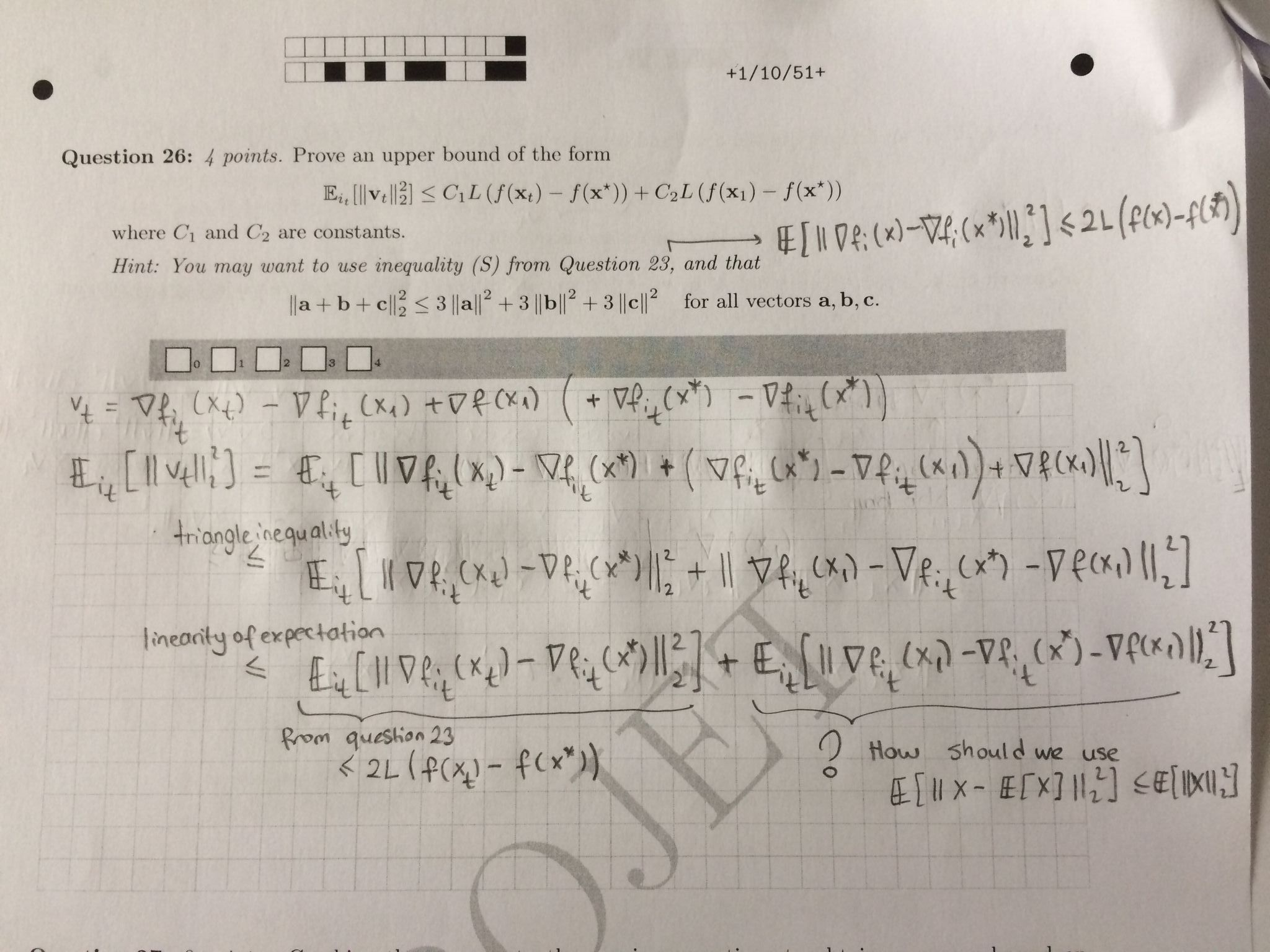### Exam 2020 Q26

Hello,

I don't understand how we obtain the second solution. What I did was to add and remove $$\nabla f(x^*)$$ from the expression, which allows to use the previous parts for the first two terms, but I don't see how to obtain the third one of the first row. Any hint on how to proceed would be very appreciated.

Top comment

$$x^*$$ is the global minimum of $$f$$ so $$\nabla f(x^*)=0$$. For $$v_t$$, you can add and remove $$\nabla f_{i_t}(x^*)$$ and add $$\nabla f(x^*)$$, then apply the inequality in the hint of question 26.

I am not the OP, but I can't understand how we obtained both solutions.
For the first solution, how the solution is not ≤ 2L but ≤4L?
Also, I don't see how the second term became the expectation of squared norm of f_it(x1) using the given hint. Could someone share his/her solution/understanding for this?
I added how I tried to solve it, but got stucked.

ThxHello,

"how the solution is not ≤ 2L but ≤4L"

because there is a 2 factor in front...

Does it help? Or I didn't understood your question?

@Anonymous said:
Hello,

"how the solution is not ≤ 2L but ≤4L"

because there is a 2 factor in front...

Does it help? Or I didn't understood your question?
I added how I approached to solve the question maybe you can look at it, cause I am still not understanding why we don't only have 2L.
Thx

@Anonymous said:
I am not the OP, but I can't understand how we obtained both solutions.
For the first solution, how the solution is not ≤ 2L but ≤4L?
Also, I don't see how the second term became the expectation of squared norm of f_it(x1) using the given hint. Could someone share his/her solution/understanding for this?
I added how I tried to solve it, but got stucked.

ThxFirst, the triangle inequality cannot be applied to the squared norm, so you should use cauchy-schwarz inequality. Thus you will finally get 4L instead of 2L.

As for the second term, the expectation is taken with respect to $$i_t$$, so you can think of $$X=\nabla f_{i_t} (x_1) - \nabla f_{i_t} (x^*)$$ and then $$\mathbb{E} X= \nabla f (x_1) - \nabla f (x^*) = \nabla f (x_1)$$. Then we can apply $$\mathbb{E} ||X-\mathbb{E}X||_2^2 \le E ||X||^2_2$$ and question 23 to get the result.

Page 1 of 1